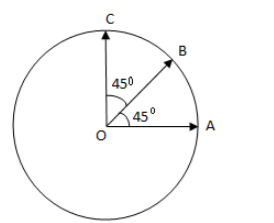Courses
Courses for Kids
Free study material
Offline Centres
MoreLast updated date: 06th Dec 2023
Total views: 281.7k
Views today: 5.81k

# Find the resultant of three vectors $\overrightarrow{OA}$, $\overrightarrow{OB}$ and $\overrightarrow{OC}$ shown in the figure given below. Radius of the circle is $R$.Verified
281.7k+ views
Hint:Vectors cannot be added simply as scalars are added because they have magnitude as well as direction. All the three vectors are in different directions. We will find their components in $X$ direction and in $Y$ direction.

Vector is a quantity which has both magnitude and direction. It is represented by an arrow whose direction is the same as that of the quantity and whose length is proportional to the quantity’s magnitude. A vector is not altered if it is displaced parallel to itself.

To add the given three vectors we will find their components in X direction and in Y direction.Let us take the direction of $\overrightarrow{OA}$ as X axis and perpendicular to this, which is the direction of $\overrightarrow{OC}$ as Y axis. Therefore, $\overrightarrow{OA}$ The vector is in X direction only and has no component in Y direction.$\overrightarrow{OC}$ has its component only in the Y direction.

Vector $\overrightarrow{OB}$ is making ${{45}^{\circ }}$angle with the X axis as well as with the Y axis.
$\left| \overrightarrow{OA} \right|=\left| \overrightarrow{OB} \right|=\left| \overrightarrow{OC} \right|=R$ (given)
$X$ components:-
Sum of vectors in $x$ direction=${{v}_{x}}$
${{v}_{x}}$= $R\cos 0+R\cos 45+R\cos 90$
$\Rightarrow {{v}_{x}}=R+\frac{R}{\sqrt{2}}+0$
$\Rightarrow {{v}_{x}}=R\left( 1+\frac{1}{\sqrt{2}} \right)$
Sum of $y$ Components=
${{v}_{x}}=R\sin 90+R\sin 45+R\sin 0 \\ \Rightarrow {{v}_{x}}=R+\frac{R}{\sqrt{2}}+0 \\ \Rightarrow {{v}_{x}}=R\left( 1+\frac{1}{\sqrt{2}} \right) \\$
$\Rightarrow \text{Resultant vector} =\sqrt{v_{x}^{2}+v_{y}^{2}}$
$\Rightarrow \text{Resultant}= \sqrt{{{\left[ R\left( 1+\frac{1}{\sqrt{2}} \right) \right]}^{2}}+{{\left[ R\left( 1+\frac{1}{\sqrt{2}} \right) \right]}^{2}}}$
$\Rightarrow \text{Resultant} =R\sqrt{2{{\left( 1+\frac{1}{\sqrt{2}} \right)}^{2}}}$
$\therefore \text{Resultant}=R\left( \sqrt{2}+1 \right)$

Hence, the resultant of three vectors is $R\left( 1+\sqrt{2} \right)$.

Note:We can use the parallelogram law of vector addition first for two vectors and then using their resultant vector with the third vector to calculate the resultant of three vectors. Do not add the vectors simply and do not forget to find their components to get the resultant vector.